top of page
###### Teaching Material

We make use of different kinds of multi-sensory teaching materials for students to visualize and experience the trial and error process.Topics

​Operations & Number Sense

• Tricks in Calculations & Operations

• Number Sense and Pattern

• Modeling problems

• Number Puzzles and Crytarithm

• Working Forward & Backward

• Multiples and Factors

• Divisibility rules

General Topics

• Pattern Ordering

• Queue Ordering

• Intervals

• Overlapping

• Age problems

• Logic and Reasoning

• Gain and Loss

• Excess and Shortage

• Ways & Routes

Measurement, Geometry, Visual Math

• Time & Date

• Measurement & Unit conversions

• Balancing

• Comparison & Equivalent Substitution

• 2D and 3D shapes

• Perimeter & Area

• Counting & Observation

• Visuo-spatial Imagination

• Distribution & Pigeonhole

• Combinations & Permutations

• Counting principles & Probability

• Venn Diagram

• Rate & Speed

• Optimization

• Fractions & Proportional Reasoning

Strategies

• Drawing a Picture or Diagram

• Using Model

• Using Deduction

• Elimination and Exclusion

• Simplification

• Finding a Number Pattern

• Finding a Geometric Pattern

• Making an Organized List

• Making a Table

• Working Backwards

• Estimation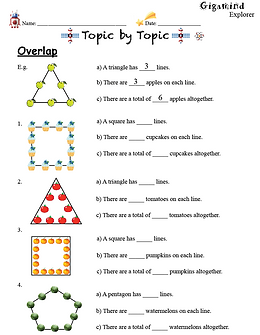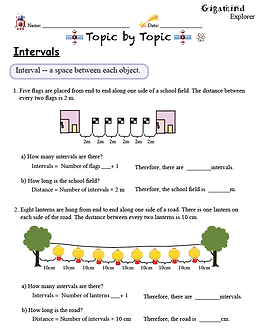## Visual representation and stimuli

A variety of Math Olympiad topics are introduced to young learners in a fun and visual way. Junior learners start from the basics and build math concepts foundation at the beginning. Our worksheets focus on using visual stimuli to help learners understand the concepts. Learners will find Math Olympiad topics interesting and different from what they normally learn at school.## Topic by Topic.                    Step by Step.

Math Olympiad topics are introduced one by one in a series of worksheets. A specific type of problem is grouped together -- from simple to complex. Students will learn strategies to solve a particular type of problem in the class. With instruction and intensive practice, learners will be able to handle that particular type of problem skillfully in the future.## Play around with numbers

If you think that math is boring, you might have never been introduced to our Math In Action series. Play around with numbers like solving a puzzle! We boost students' ability to think fast, on their feet, and act it out.

You will find a lot of fun and challenges here. Like Number puzzles, Number patterns, Crytarihm, Sudoku, Guess what number I am ...and more!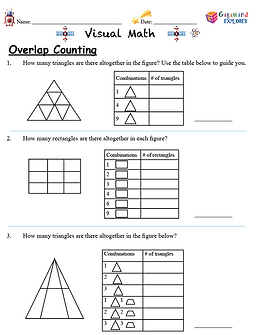## Inspiration for creativity and imaginative thinking

Many educators neglect the visual aspect of math. In fact, visual math plays a great part in our daily lives and can be applied to many professional fields. We boost students' ability to observe, imagine, and create.

Concepts and skills

• Counting and observation

• Shape and geometric pattern

• Symmetry, cutting and folding

• Slide, turn and flip

• Position and direction

• Neighbor coloring

• The Euler path## Numbers and Operations

Not having enough practice on basic operations? Being pushed away by numbers? Feeling uncomfortable and not confident enough?

This series is a booster for you.

In this series, you will learn and practice:

• Digit and place value

• Properties of numbers

• Tricks in four operations

• Fun Calculation and Difference Application

• Multiples and factors

• Divisibility rules and remainders

• Multiple steps problem solving

• Exponent and square root

• Positives and negatives on a number line

• Fractions and decimals

• Ratio and Percentage

• Basic statistics

• Algebra

• Bases other than 10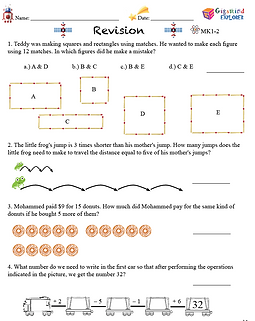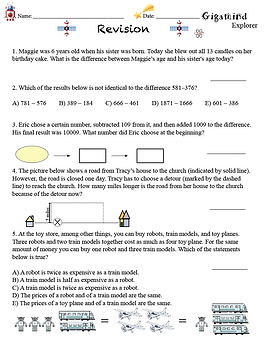## Apply the knowledge and skills to the problems

A variety of Math Olympiad problems and conventional school curriculum problems are mixed and presented in the revision series. Intermediate learners have adequate math knowledge of their age and good reading skills to solve the problems. Learners will practice how to handle the problems by choosing a strategy skillfully and apply it to the problems.## It's time to take a challenge.

Advanced learners will start practicing more challenging problems that appear in national math contests and competitions. At this stage, learners should be familiar with the Math Olympiad topics and able to apply strategies and skills to different types of problems. Learners will practice sample questions from previous exams and competitions.

## Not ready for the challenges? Here are more basic practices.Additional but essential practice -- A solid foundation for the four operations helps boost the speed and accuracy for problem-solving. This series of workbook can help 1st and 2nd graders build a conceptual understanding of multiplication and division, fractions, and algebra.

bottom of page